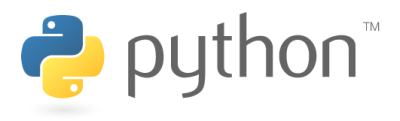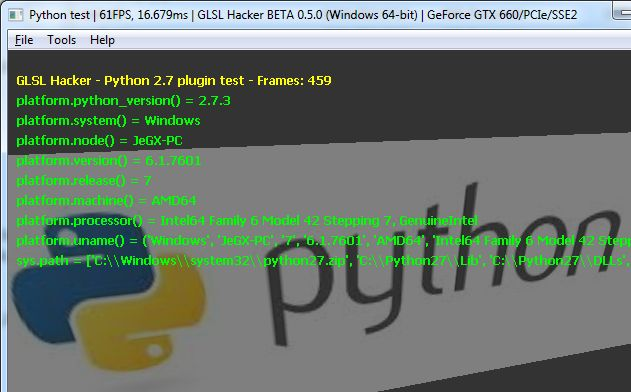# Python Primer for the ImpatientPython is one of the scripting languages supported by GLSL Hacker. Here is a quick introduction to the essential notions and syntax of Python programming language. All following notions are general and are not specific to GLSL Hacker. Thanks to this primer, you will be able to quickly tweak and hack GLSL Hacker demos. GLSL Hacker 0.5.0 is available with a Python 2.7 plugin.

The reference manual of Python 2 can be found HERE.

This primer does not cover advanced topics like object oriented programming. It follows the same line than Lua Primer fro the Impatient: providing a quick way to read and write basic Python programs or scripts.

Single line comment: #

```# this is a single line comment in Python
```

Multi-line comment with triple quotes: “””

```"""
this is a
multi-line
comment in Python
"""
```

## 2 – Variables

Variables in Python have a type but there is no type declaration. Common types are numbers (float, integer), strings, lists, tuples and dictionnaries. Variables defined in a function have local scope while Variables defined outside functions have global scope.

```num_vertices = 0 # integer variable
width = 10.25 # float variable
mesh_name = "my_kool_mesh" # string variable
```

Tuples use parentheses while lists use brackets. Tuples can’t be updated, their size can’t be changed while lists can be updated, elements can be added or removed.

```shader_tuple = ('Vertex', 'Fragment', 'Geometry') # tuple

program_list = ['prog01', 'prog02', 'prog03'] # list
program_list.append('prog04')
program_list.append('prog05')
```

Dictionnaries is a kind of hash table and are made up of pairs of key:value. The key is usually a number or a string.

```node_dict = {} # dictionnary
node_dict['node01'] = 100
node_dict['node02'] = 101
node_dict['node03'] = 102
```

To sum up:

```my_tuple = ()
my_list = []
my_dict = {}
```

You can convert a type to another type with float(), int(), str(), tuple() or dict():

```x = 10
x_str = str(x)
```

To concatenate strings, just use the + operator:

```space = " "
app_name = "GLSL" + space + "Hacker"
```

## 3 – Indentation

Unlike C, PHP or Lua, Python does not have braces to delimit blocks of code like functions or tests. To delimit blocks of code, Python uses indentation and indentation is severely inspected by Python, The smallest difference in indentation leads to fatal compilation error! So be careful with indentation and use a correct text editor to handle indentation properly.

## 4 – Functions

Functions in Python can take multiple arguments and can return multiple results.

```def myKoolFunc(a, b, c):
# indentation!!!
# Do something useful with a, b and c:
sum = a+b+c
avg = sum/3
return sum, avg

x, y = myKoolFunc(1, 2, 3)
```

## 5 – Operators

Comparison: The comparison operators are the same than in C language:

• equal: (a == b)
• not equal: (a != b)
• greater than: (a > b)
• greater than or equal: (a >= b)
• lesser than: (a < b)
• lesser than or equal: (a <= b)

Logical:

• and: (a and b)
• or: (a or b)

Bitwise:

• binary AND: (a & b)
• binary OR: (a | b)
• binary left shift: a << b
• binary right shift: a >> b
• binary XOR: (a ^ b)
• binary complement: ~a

## 6 – Control Structures

Conditional tests:

```if (a == x):
# do something
elif (a == y):
# do something
else:
# do something
```

Loops

for:

```for i in range(0, 10):
# do something

for i in "GLSL Hacker":
print(i) # print current letter

for v in range(len(vertices_list)):
Update_Position(v)

```

while:

```i=0
while (i < 10):
# do something
i += 1
```

## 7 - Built-in functions

Python comes with a ton of modules and that's one of the strength of Python:

Fans of Python use the phrase batteries included to describe the standard library, which covers everything from asynchronous processing to zip files. The language itself is a flexible powerhouse that can handle practically any problem domain. Build your own web server in three lines of code. Build flexible data-driven code using Python's powerful and dynamic introspection capabilities and advanced language features such as meta-classes, duck typing and decorators.

There are so many modules and functions in Python standard library and I'm not going to list them here. Nonetheless, I can give you an example of the use of platform module to get the version of Python. To use a module, use the import keyword:

```import platform
py_version = str(platform.python_version())
```

There is a demo in GLSL Hacker Code Sample Pack that shows the use of the platform module:Like in Lua, Python has a math library:

```import math
```import random